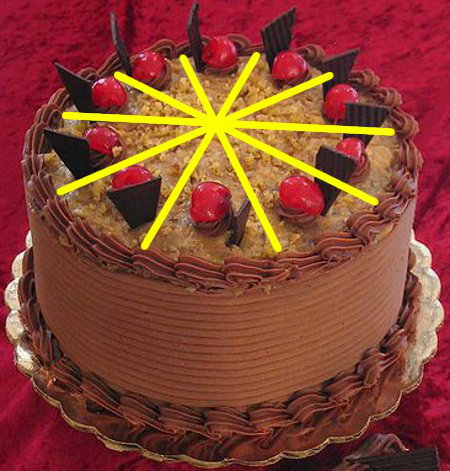# Fractions To DecimalsThis cake has 10 slices. One slice is one-tenth or 110 of the cake. Two slices are two-tenths or 210 of the cake.

To change common fractions to decimal fractions, divide the numerator (on the top) by the denominator (on the bottom).

## Terminating Decimals to Learn

 COMMON FRACTION DECIMAL FRACTION 1⁄10 0.1 2⁄10 = 2⁄5 0.2 3⁄10 0.3 4⁄10 = 2⁄5 0.4 5⁄10 = 1⁄2 0.5 6⁄10 = 3⁄5 0.6 7⁄10 0.7 8⁄10 = 4⁄5 0.8 9⁄10 0.9 1⁄4 0.25 3⁄4 0.75 1⁄8 0.125 3⁄8 0.375 5⁄8 0.625 7⁄8 0.875

## Recurring Decimals to Learn

 COMMON FRACTION DECIMAL FRACTION 1⁄3 0.3 2⁄3 0.6 1⁄6 0.16 1⁄9 0.1 1⁄11 0.09

## Questions

Use a calculator to change these common fractions to decimal fractions.
Divide the top number by the bottom number.
Round each answer to two decimal places.

Q1. 37
Q2. 423
Q3. 513
Q4. 67
Q5. 713

A1. 0.43
A2. 0.17
A3. 0.38
A4. 0.86
A5. 0.54

## Maths Fun - Recurring Decimals

17 = 0.142857 142857 142857...

117 = 0.0588235294117647 0588235294117647 0588235294117647 ...

127 = 0.037 037 037...

137 = 0.027 027 027...

Use a computer calculator to find the repeating part in these decimals: 19, 29, 39, 49, 59, 69, 79, 89, 111, 211, 311 and so on. What do you notice?